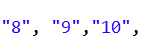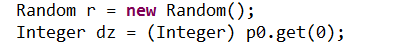import java.util.ArrayList;
import java.util.Collections;
import java.util.HashMap;
import java.util.Random;
import java.util.Set;

//    张三，李四，王五三个人打斗地主，三人约定，洗牌后，
//    随机抽取一张"明牌"并夹在中间；然后依次抓牌，谁抓到这张便自动作为地主，并收取最后三张。
//    要求：请用程序实现这一过程，最后打印地主名，以及三个人的牌(要求排序)；
//    思路：
//        1.定义一个Map集合存储一副扑克牌；List存储编号；
//        2.洗牌；
//        3.随机抽取一个索引(该值必须在倒数三张之前)，用于表示"明牌"，在发牌
//        时谁抓到这一张便作为"地主"；
//        4.依次给三个人(张三，李四，王五)发牌，并监督谁作为地主；地主自动收取最后三张。
//      5.打印地主名；
//      6.最后以排序后的方式打印每个人手里的牌
public class Demo03 {
public static void main(String[] args) {
HashMap map = new HashMap();
// 花色
ArrayList flower = new ArrayList();
// 数字
ArrayList number = new ArrayList();
Collections.addAll(number, "3", "4", "5", "6", "7", "8", "10", "J", "Q", "K", "A", "2");
Integer a = 1;
for (String number1 : number) {
for (String flower1 : flower) {
String s = flower1 + number1;
map.put(a++, s);
}
}
map.put(53, "小☆");
map.put(54, "大★");
System.out.println(map);

Set keySet = map.keySet();
ArrayList list = new ArrayList();
for (Integer integer : keySet) {
}
System.out.println(list);

Collections.shuffle(list);

System.out.println(list);

ArrayList p1 = new ArrayList();
ArrayList p2 = new ArrayList();
ArrayList p3 = new ArrayList();
ArrayList p0 = new ArrayList();
System.out.println(list.size());
for (int i = 0; i < list.size(); i++) {
if (i >= 51) {
} else {
if (i % 3 == 0) {
} else if (i % 3 == 1) {
} else if (i % 3 == 2) {
}
}

}
System.out.println(p1);
System.out.println(p2);
System.out.println(p3);
System.out.println(p0);
Random r = new Random();
Integer dz = null;
while ((p0.contains(dz)) ){
dz = r.nextInt(54) + 1;

}

dz(p1, dz, p0);
dz(p2, dz, p0);
dz(p3, dz, p0);

ArrayList player1 = new ArrayList();
ArrayList player2 = new ArrayList();
ArrayList player3 = new ArrayList();

for (Integer integer : p1) {
}
for (Integer integer : p2) {
}
for (Integer integer : p3) {
}
System.out.println("张三=" + player1);
System.out.println("李四=" + player2);
System.out.println("王五=" + player3);

}

public static void dz(ArrayList p, Integer dz, ArrayList p0) {
System.out.println("试试");
int count = -1;
for (int i = 0; i < p.size(); i++) {
if (dz.equals(p.get(i))) {
count = i;
}

}
if (count != -1) {
for (int j = 0; j < p0.size(); j++) {
System.out.println("试试1");
System.out.println(p + "");
}
}
if (count == -1) {
return;
}

}

}

7个回答

Collections.addAll(number, "3", "4", "5", "6", "7", "8", "10", "J", "Q", "K", "A", "2");package csdn;

import java.util.ArrayList;
import java.util.Collections;
import java.util.HashMap;
import java.util.List;
import java.util.Map;
import java.util.Random;
import java.util.Set;
//张三，李四，王五三个人打斗地主，三人约定，洗牌后，
//随机抽取一张"明牌"并夹在中间；然后依次抓牌，谁抓到这张便自动作为地主，并收取最后三张。
//要求：请用程序实现这一过程，最后打印地主名，以及三个人的牌(要求排序)；
//思路：
// 1.定义一个Map集合存储一副扑克牌；List存储编号；
// 2.洗牌；
// 3.随机抽取一个索引(该值必须在倒数三张之前)，用于表示"明牌"，在发牌
// 时谁抓到这一张便作为"地主"；
// 4.依次给三个人(张三，李四，王五)发牌，并监督谁作为地主；地主自动收取最后三张。
//5.打印地主名；
//6.最后以排序后的方式打印每个人手里的牌
public class Demo3 {
public static void main(String[] args) {
Map map = new HashMap();
// 花色
List flower = new ArrayList();
// 数字
List number = new ArrayList();
Collections.addAll(number, "3", "4", "5", "6", "7", "8", "9","10", "J", "Q", "K", "A", "2");
Integer a = 1;
for (String number1 : number) {
for (String flower1 : flower) {
String s = flower1 + number1;
map.put(a++, s); //生成扑克牌
}
}
map.put(53, "小☆");
map.put(54, "大★");
System.out.println(map);

``````        Set<Integer> keySet = map.keySet();
List list = new ArrayList();
for (Integer integer : keySet) {
}
System.out.println(list);

Collections.shuffle(list);//打乱编号，相当于洗牌

System.out.println(list);

List<Integer> p1 = new ArrayList();
List<Integer> p2 = new ArrayList();
List<Integer> p3 = new ArrayList();
List p0 = new ArrayList();
System.out.println(list.size());
for (int i = 0; i < list.size(); i++) {//list的size是54
if (i >= 51) {
} else {
if (i % 3 == 0) {
} else if (i % 3 == 1) {
} else if (i % 3 == 2) {
}
}
}
System.out.println(p1);
System.out.println(p2);
System.out.println(p3);
System.out.println(p0);

/*List<Integer> p4=new ArrayList();
System.out.println(p4);
System.out.println(p4.size());*/
Random r = new Random();
Integer dz = (Integer) p0.get(0);
while ((p0.contains(dz)) ){
dz = r.nextInt(54) + 1;
}
System.out.println(dz);
dz(p1, dz, p0);
dz(p2, dz, p0);
dz(p3, dz, p0);
``````

//
List player1 = new ArrayList();
List player2 = new ArrayList();
List player3 = new ArrayList();

``````        for (Integer integer : p1) {
}
for (Integer integer : p2) {
}
for (Integer integer : p3) {
}
System.out.println("张三=" + player1);
System.out.println("李四=" + player2);
System.out.println("王五=" + player3);
}

public static void dz(List<Integer> p1, Integer dz, List p0) {
System.out.println("试试");
int count = -1;
for (int i = 0; i < p1.size(); i++) {
if (dz.equals(p1.get(i))) {
count = i;
}

}
if (count != -1) {
for (int j = 0; j < p0.size(); j++) {
System.out.println("试试1");
System.out.println(p1 + "");
}
}
if (count == -1) {
return;
}
}
}
``````

package com.RegularExpression;

import java.util.ArrayList;
import java.util.Collections;
import java.util.HashMap;
import java.util.List;
import java.util.Map;
import java.util.Random;
import java.util.Set;

/***

• @author: Libra
• Date: 2017年5月12日 上午9:28:42
• Version: 1.0
• @Description: 斗地主
*/
public class FightLandlord {

/** 一整副扑克牌(以Map形式保存) /
private static Map map = null;
/
* 编号(以List形式保存) */
private static List list ;

public static void main(String[] args) {
//拿到扑克牌
map = getPoker();
//每张扑克牌对应的编号
list = new ArrayList();
Set keySet = map.keySet();
for (Integer integer : keySet) {
}

``````    /** 洗牌  */
Collections.shuffle(list);

/** 发牌以及底牌  */
//张三
List<String> p1 = new ArrayList<String>();
//李四
List<String> p2 = new ArrayList<String>();
//王五
List<String> p3 = new ArrayList<String>();
//底牌
List<String> p0 = new ArrayList<String>();

for (int i = 0; i < list.size(); i++) {
if (i >= 51) {
} else {
if (i % 3 == 0) {
} else if (i % 3 == 1) {
} else if (i % 3 == 2) {
}
}
}

/** 抢地主  */
Random r = new Random();
String dz = String.valueOf((r.nextInt(54) + 1));
while ((p0.contains(dz)) ){
break;
}

List<String> dz1 = dz(p1, dz, p0);
List<String> dz2 = dz(p2, dz, p0);
List<String> dz3 = dz(p3, dz, p0);
if(null != dz1){
System.out.println("本轮的地主为：" + "张三");
}else if(null != dz2){
System.out.println("本轮的地主为：" + "李四");
}else if(null != dz3){
System.out.println("本轮的地主为：" + "王五");
}

List<String> player1 = new ArrayList<String>();
List<String> player2 = new ArrayList<String>();
List<String> player3 = new ArrayList<String>();

for (String integer : p1) {
}
for (String integer : p2) {
}
for (String integer : p3) {
}
System.out.println("张三=" + player1);
System.out.println("李四=" + player2);
System.out.println("王五=" + player3);
}
``````

/**

• @method 整合扑克牌
• @return 一整副扑克牌 */ private static Map getPoker(){ Map poker = new HashMap(); // 花色 ArrayList flower = new ArrayList(); Collections.addAll(flower, "♣", "♦", "♠", "♥"); // 数字 ArrayList number = new ArrayList(); Collections.addAll(number, "3", "4", "5", "6", "7", "8", "9","10", "J", "Q", "K", "A", "2"); Integer a = 1; for (String number1 : number) { for (String flower1 : flower) { String s = flower1 + number1; poker.put(a++, s); } } poker.put(53, "小☆"); poker.put(54, "大★"); return poker; }

/**

• @method 抢地主
• @param member
• @param dz 地主牌所在的编号
• @param bot
*/
public static List dz(List member, String dz, List bot) {
int count = -1;
for (int i = 0; i < member.size(); i++) {
if (dz.equals(member.get(i))) {
count = i;
}
}

if (count != -1) {
for (int j = 0; j < bot.size(); j++) {
}
}else{
return null;
}

return member;
}

}

package test;

import java.util.ArrayList;
import java.util.Collections;
import java.util.HashMap;
import java.util.List;
import java.util.Map;
import java.util.Random;
import java.util.Set;

// 张三，李四，王五三个人打斗地主，三人约定，洗牌后，
// 随机抽取一张"明牌"并夹在中间；然后依次抓牌，谁抓到这张便自动作为地主，并收取最后三张。
// 要求：请用程序实现这一过程，最后打印地主名，以及三个人的牌(要求排序)；
// 思路：
// 1.定义一个Map集合存储一副扑克牌；List存储编号；
// 2.洗牌；
// 3.随机抽取一个索引(该值必须在倒数三张之前)，用于表示"明牌"，在发牌
// 时谁抓到这一张便作为"地主"；
// 4.依次给三个人(张三，李四，王五)发牌，并监督谁作为地主；地主自动收取最后三张。
// 5.打印地主名；
// 6.最后以排序后的方式打印每个人手里的牌
public class Demo03 {
public static void main(String[] args) {
Map map = new HashMap();
// 花色
List flower = new ArrayList();
// 数字
List number = new ArrayList();
Collections.addAll(number, "3", "4", "5", "6", "7", "8", "9","10", "J", "Q", "K", "A", "2");
Integer a = 1;
for (String number1 : number) {
for (String flower1 : flower) {
String s = flower1 + number1;
map.put(a++, s);
}
}
map.put(53, "小☆");
map.put(54, "大★");
System.out.println(map);
Set keySet = map.keySet();
List list = new ArrayList();
for (Integer integer : keySet) {
}
System.out.println(list);
Collections.shuffle(list);
System.out.println(list);
List p1 = new ArrayList();
List p2 = new ArrayList();
List p3 = new ArrayList();
List p0 = new ArrayList();
System.out.println(list.size());
for (int i = 0; i < list.size(); i++) {
if (i >= 50) {
} else {
if (i % 3 == 0) {
} else if (i % 3 == 1) {
} else if (i % 3 == 2) {
}
}
}
System.out.println(p1);
System.out.println(p2);
System.out.println(p3);
System.out.println(p0);
Random r = new Random();
Integer dz = 0;
Integer dzNext = 0;//名牌的下一张，谁抓到这张 谁就有名牌；

// while (p0.contains(dz) ){
dz = p0.get(r.nextInt(p0.size()));// 名牌
dzNext = dz + 1;
// }

``````    dz(p1, dzNext, p0);
dz(p2, dzNext, p0);
dz(p3, dzNext, p0);
List<String> player1 = new ArrayList<String>();
List<String> player2 = new ArrayList<String>();
List<String> player3 = new ArrayList<String>();
List<String> last = new ArrayList<String>();
for (Integer integer : p1) {
}
for (Integer integer : p2) {
}
for (Integer integer : p3) {
}
for (Integer integer : p0) {
}
System.out.println("张三=" + player1 + "  " + p1);
System.out.println("李四=" + player2 + "  " + p2);
System.out.println("王五=" + player3 + "  " + p3);
System.out.println("last=" + player3 + p0);
System.out.println("名牌=" + dz + "  " + map.get(dz));
}
public static void dz(List<Integer> p, Integer dz, List<Integer> p0) {
System.out.println("试试");
int count = -1;
for (int i = 0; i < p.size(); i++) {
if (dz.equals(p.get(i)-1)) {
count = i;
}

}
System.out.println("count ="+count);
if (count != -1) {
for (int j = 0; j < p0.size(); j++) {
System.out.println("试试1");
System.out.println(p + "");
}
}
if (count == -1) {
return;
}
}
``````

}VS+MFC+opengl系统开发的问题？（初学）

![图片说明](https://img-ask.csdn.net/upload/201909/25/1569378289_314255.png)![图片说明](https://img-ask.csdn.net/upload/201909/25/1569378350_626790.png) 批量数据插入CODE表 解析code.csv文件批量插入CODE[PFPN]） 插入DB时，做简单检查：主键(前三个栏位)中任何栏位不允许为空，不符合条件的节点不处理，但需要记录不符合条件的记录的总数，在返回结果中体现。 出现重复记录时不要报错而是更新，最终返回结果需要返回更新和插入的记录条数。 这是数据库表的基本结构，前三个栏位是主键 INSERT INTO cms.ccode (CODE_CATE, CODE, CODE_EXT, SUBITEM, CODE_DSC, EXT_1, EXT_2, EXT_3, EXT_4, EXT_5) VALUES('PFPN', '0002', 'P101TCAWA0Z', 'P101CCAW70Z', '10.1', '1410901-A1', 'N1011', 'C101NWT3', 'P101TCAWA1', 'M01');
C语言 如何编写将字符串及数字转化成十六进制的密码集？急急急！感谢！

Mac Python3.7, pyinstaller 生成的exe文件无法执行，报错Failed to execute script pyi_rth__tkinter

Python编程驾驶飞船游戏。
# python新手小白，在学《Python编程从入门到实践》 编写‘驾驶飞船’游戏## 主程序 alien_invasion ： ``` import sys import pygame import game_functions as gf from settings import Settings from ship import Ship def run_game(): pygame.init() ai_settings = Settings() screen = pygame.display.set_mode( (ai_settings.screen_width,ai_settings.screen_height)) ship = Ship(screen) pygame.display.set_caption("Alien Invasion") while True: gf.check_events(ship) gf.update_screen(ai_settings,screen,ship) pygame.display.flip() run_game() ``` ## 调用的game_functions： ``` import sys import pygame def check_events(ship): for event in pygame.event.get(): if event.type == pygame.QUIT: sys.exit() elif event.type == pygame.KEYDOWM: if event.key == pygame.K_RIGHT: ship.rect.centerx += 1 def update_screen(ai_settings,screen,ship): screen.fill(ai_settings.bg_color) ship.blitme() ``` 运行的时候，显示调用的函数有问题，错误代码： AttributeError module ‘pygame’ have no attribute ‘KEYDOWN’,翻来覆去不知道为啥，请路过的大神解答，指点迷津
java 实习 ，现在放暑假了，我想提前找实习的，投了十多份除了培训的没人理会我，想问下前辈该怎么办
java 部分我有学springmvc springboot mybatis，目前在学redis， 前端部分也有学 vue框架 会前后端分离 有写过电商网站 http://47.107.36.244/cxp 想问下前辈还有哪些是我现在应该掌握的没学的，还有找不到实习的该怎么办好

Parameter index out of range (2 > number of parameters, which is 1)

java中的枚举问题中的变量

JAVA 自定义类导入报错的问题。。。。

Dart这么吊，java怎么办？

Java学习的正确打开方式

linux系列之常用运维命令整理笔录

Python十大装B语法
Python 是一种代表简单思想的语言，其语法相对简单，很容易上手。不过，如果就此小视 Python 语法的精妙和深邃，那就大错特错了。本文精心筛选了最能展现 Python 语法之精妙的十个知识点，并附上详细的实例代码。如能在实战中融会贯通、灵活使用，必将使代码更为精炼、高效，同时也会极大提升代码B格，使之看上去更老练，读起来更优雅。

2019年11月中国大陆编程语言排行榜
2019年11月2日，我统计了某招聘网站，获得有效程序员招聘数据9万条。针对招聘信息，提取编程语言关键字，并统计如下： 编程语言比例 rank pl_ percentage 1 java 33.62% 2 cpp 16.42% 3 c_sharp 12.82% 4 javascript 12.31% 5 python 7.93% 6 go 7.25% 7 p...

《奇巧淫技》系列-python！！每天早上八点自动发送天气预报邮件到QQ邮箱

SQL-小白最佳入门sql查询一

JavaScript 为什么能活到现在？

Python 编程开发 实用经验和技巧
Python是一门很灵活的语言，也有很多实用的方法，有时候实现一个功能可以用多种方法实现，我这里总结了一些常用的方法和技巧，包括小数保留指定位小数、判断变量的数据类型、类方法@classmethod、制表符中文对齐、遍历字典、datetime.timedelta的使用等，会持续更新......

“狗屁不通文章生成器”登顶GitHub热榜，分分钟写出万字形式主义大作

《程序人生》系列-这个程序员只用了20行代码就拿了冠军

11月8日，由中国信息通信研究院、中国通信标准化协会、中国互联网协会、可信区块链推进计划联合主办，科技行者协办的2019可信区块链峰会将在北京悠唐皇冠假日酒店开幕。 　　区块链技术被认为是继蒸汽机、电力、互联网之后，下一代颠覆性的核心技术。如果说蒸汽机释放了人类的生产力，电力解决了人类基本的生活需求，互联网彻底改变了信息传递的方式，区块链作为构造信任的技术有重要的价值。 　　1...